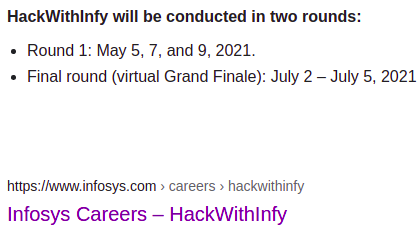# Post Hackwithinfy discussion

1. Given three integers L,R,X find how many intergers k in range L to R satisfy the below condition
k&X=X , where & is bitwise and
Constraints
1<= L <= R <=1e18

input
L=2 R=3 X=2
output
2

2. You are given a 2d matrix of N x N and an integer K . Your task is to turn this matrix into an almost equal matrix
A matrix is said to be almost equal if for any 2 adjacent elements their absolute difference is <=k
Adjacent cell of (r,c) are (r+1,c) and (r,c+1)
Calculate mimimum number of operation required to make the matrix almost equal

Constraint
1<= N <=500
1<= K <=10
1<= Matix[i][j] <=1000

Input
2
4
1 7
7 13

output
8

explanation
equal matrix is
5 9
9 13

The first problem is pretty trivial, I mean you can always run a digit dp to get a logarithmic time solution, but I’m pretty sure there exists a neat combinatorial solution to this. It’d be great if someone can brief that solution once, it’s not striking to me right now. For the time being, I’ll attach my dp solution, just in case anyone wanna have a look(do let me know if this fails some case I haven’t tested it rigorously).

DIGIT DP SOLUTION
#include "bits/stdc++.h"
#define int long long
using namespace std ;
signed main(){
int l,r,x ;
cin >> l >> r  >> x  ;
auto get=[&](int N){
if(N<x)
return 0LL ;
int N1=N,d=1,x1=x,msb=0,c=0 ;
while(x1){
if(N1%2==1 && x1%2==0)
d<<=1 ;
c+=((x1&1)==0) ;x1>>=1 ;msb++ ;
N1>>=1 ;
}
vector<int>dp(62) ; dp=1 ;
for(int i=1,j=0;i<=msb;i++){
if((N>>(i-1))%2==0 && ((x>>(i-1))&1)==1)
dp[i]=0 ;
else if((((N>>(i-1))&1)^((x>>(i-1))&1)==0))
dp[i]=dp[i-1] ;
else
dp[i]=dp[i-1]+(1LL<<j) ;
j+=(((x>>(i-1))&1)==0) ;
}
for(int i=msb,j=0;i<62;i++,j++)
dp[i+1]=dp[i]+((N>>i)&1)*(1LL<<(j+c)) ;
return dp ;
}   ;
cout << get(r)-get(l-1) ;
}


PS: Can anyone please confirm if this is from some ongoing contest or not?

1 LikeYup, the contest is over.

1 Like

Oh, this the same contest that you pointed out earlier, thanks!

Again thanks for help. Any idea for 2 question i am very confused because for any (r,c) if there is bigger number at (r+2,c) or (r,c+2) then we have to increase (r+1,c) or (r,c+1) then we have to again update (r,c) if there is smaller number . I can’t think of any efficient algorithm

Btw may I know the reason why are you posting from this account instead of @cortex790 ? I hope you don’t want to lose the progress you made on that account, do you ?

I got bored with cp just couldn’t see any increase in ranking so i stopped using that account. Btw how you got to know about my other account.

Kindly delete one of the accounts if you don’t want to be penalized.

My post will be deleted then. A lot of people are using 2 account they make editorial video using other account then why i am being penalizedI think 2nd problem can be solved by DP if we approach it from bottom right and move to top left
We maintain a 3D dp array, and for any step (i,j)
dp[i][j][x]=min(dp[i][j+1][x_1] \forall x_1 \in[x-k,x+k] ) + min(dp[i+1][j][x_2] \forall x_2 \in[x-k,x+k]) + x-mat[i][j]
x \geq mat[i][j]
This, however is a painfully slow solution O(N^2.max(a[i]).log(K)) \approx O(N^2*max(a[i])) and I m not sure it will pass under 1 sec.

Suggestions welcome.

How is an operation defined though? I didn’t get that.

You have to add 1 to (r,c) or (r+1,c) or (r,c+1) cell such that absolute difference between {(r,c),(r+1,c)} and {(r,c), (r,c+1)} is <=k

This exact same question is asked in google kickstart 2021 Round 1,
I solved it with Max heap.

Bruh I practiced all rounds except round ADon’t Know why anyone who can solve 2200+ rated cf problem will join Infosys . My Set involved all dp problems could only solve 1 problem and other participants got atleast 1 cf A|B level questions , felt a bit partiality. Btw i edited neal wu’s solution is this correct?